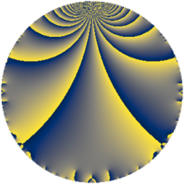# Properties

 Label 2151.2.a.aLevel $2151$ Weight $2$ Character orbit 2151.a Self dual yes Analytic conductor $17.176$ Analytic rank $1$ Dimension $2$ CM no Inner twists $1$

# Related objects

## Newspace parameters

 Level: $$N$$ $$=$$ $$2151 = 3^{2} \cdot 239$$ Weight: $$k$$ $$=$$ $$2$$ Character orbit: $$[\chi]$$ $$=$$ 2151.a (trivial)

## Newform invariants

 Self dual: yes Analytic conductor: $$17.1758214748$$ Analytic rank: $$1$$ Dimension: $$2$$ Coefficient field: $$\Q(\sqrt{5})$$ Defining polynomial: $$x^{2} - x - 1$$ Coefficient ring: $$\Z[a_1, a_2]$$ Coefficient ring index: $$1$$ Twist minimal: no (minimal twist has level 717) Fricke sign: $$1$$ Sato-Tate group: $\mathrm{SU}(2)$

## $q$-expansion

Coefficients of the $$q$$-expansion are expressed in terms of $$\beta = \frac{1}{2}(1 + \sqrt{5})$$. We also show the integral $$q$$-expansion of the trace form.

 $$f(q)$$ $$=$$ $$q + ( -1 - \beta ) q^{2} + 3 \beta q^{4} + ( -1 + 2 \beta ) q^{5} + ( -1 - 4 \beta ) q^{8} +O(q^{10})$$ $$q + ( -1 - \beta ) q^{2} + 3 \beta q^{4} + ( -1 + 2 \beta ) q^{5} + ( -1 - 4 \beta ) q^{8} + ( -1 - 3 \beta ) q^{10} -3 q^{11} + ( 2 - 2 \beta ) q^{13} + ( 5 + 3 \beta ) q^{16} + ( 3 - 2 \beta ) q^{17} + ( 6 + 3 \beta ) q^{20} + ( 3 + 3 \beta ) q^{22} + 2 \beta q^{23} + 2 \beta q^{26} + ( -3 + 2 \beta ) q^{29} + ( 5 - 6 \beta ) q^{31} + ( -6 - 3 \beta ) q^{32} + ( -1 + \beta ) q^{34} + ( 6 - 6 \beta ) q^{37} + ( -7 - 6 \beta ) q^{40} + ( 2 - 2 \beta ) q^{41} + ( -6 + 4 \beta ) q^{43} -9 \beta q^{44} + ( -2 - 4 \beta ) q^{46} + 4 \beta q^{47} -7 q^{49} -6 q^{52} + ( -8 - 2 \beta ) q^{53} + ( 3 - 6 \beta ) q^{55} + ( 1 - \beta ) q^{58} + ( 6 - 6 \beta ) q^{59} -7 q^{61} + ( 1 + 7 \beta ) q^{62} + ( -1 + 6 \beta ) q^{64} + ( -6 + 2 \beta ) q^{65} + ( 1 - 6 \beta ) q^{67} + ( -6 + 3 \beta ) q^{68} -12 q^{71} + ( -2 + 6 \beta ) q^{73} + 6 \beta q^{74} + ( -6 + 2 \beta ) q^{79} + ( 1 + 13 \beta ) q^{80} + 2 \beta q^{82} + ( 11 + 4 \beta ) q^{83} + ( -7 + 4 \beta ) q^{85} + ( 2 - 2 \beta ) q^{86} + ( 3 + 12 \beta ) q^{88} -4 \beta q^{89} + ( 6 + 6 \beta ) q^{92} + ( -4 - 8 \beta ) q^{94} + ( -6 + 10 \beta ) q^{97} + ( 7 + 7 \beta ) q^{98} +O(q^{100})$$ $$\operatorname{Tr}(f)(q)$$ $$=$$ $$2q - 3q^{2} + 3q^{4} - 6q^{8} + O(q^{10})$$ $$2q - 3q^{2} + 3q^{4} - 6q^{8} - 5q^{10} - 6q^{11} + 2q^{13} + 13q^{16} + 4q^{17} + 15q^{20} + 9q^{22} + 2q^{23} + 2q^{26} - 4q^{29} + 4q^{31} - 15q^{32} - q^{34} + 6q^{37} - 20q^{40} + 2q^{41} - 8q^{43} - 9q^{44} - 8q^{46} + 4q^{47} - 14q^{49} - 12q^{52} - 18q^{53} + q^{58} + 6q^{59} - 14q^{61} + 9q^{62} + 4q^{64} - 10q^{65} - 4q^{67} - 9q^{68} - 24q^{71} + 2q^{73} + 6q^{74} - 10q^{79} + 15q^{80} + 2q^{82} + 26q^{83} - 10q^{85} + 2q^{86} + 18q^{88} - 4q^{89} + 18q^{92} - 16q^{94} - 2q^{97} + 21q^{98} + O(q^{100})$$

## Embeddings

For each embedding $$\iota_m$$ of the coefficient field, the values $$\iota_m(a_n)$$ are shown below.

For more information on an embedded modular form you can click on its label.

Label $$\iota_m(\nu)$$ $$a_{2}$$ $$a_{3}$$ $$a_{4}$$ $$a_{5}$$ $$a_{6}$$ $$a_{7}$$ $$a_{8}$$ $$a_{9}$$ $$a_{10}$$
1.1
 1.61803 −0.618034
−2.61803 0 4.85410 2.23607 0 0 −7.47214 0 −5.85410
1.2 −0.381966 0 −1.85410 −2.23607 0 0 1.47214 0 0.854102
 $$n$$: e.g. 2-40 or 990-1000 Significant digits: Format: Complex embeddings Normalized embeddings Satake parameters Satake angles

## Atkin-Lehner signs

$$p$$ Sign
$$3$$ $$-1$$
$$239$$ $$-1$$

## Inner twists

This newform does not admit any (nontrivial) inner twists.

## Twists

By twisting character orbit
Char Parity Ord Mult Type Twist Min Dim
1.a even 1 1 trivial 2151.2.a.a 2
3.b odd 2 1 717.2.a.c 2

By twisted newform orbit
Twist Min Dim Char Parity Ord Mult Type
717.2.a.c 2 3.b odd 2 1
2151.2.a.a 2 1.a even 1 1 trivial

## Hecke kernels

This newform subspace can be constructed as the intersection of the kernels of the following linear operators acting on $$S_{2}^{\mathrm{new}}(\Gamma_0(2151))$$:

 $$T_{2}^{2} + 3 T_{2} + 1$$ $$T_{5}^{2} - 5$$

## Hecke characteristic polynomials

$p$ $F_p(T)$
$2$ $$1 + 3 T + T^{2}$$
$3$ $$T^{2}$$
$5$ $$-5 + T^{2}$$
$7$ $$T^{2}$$
$11$ $$( 3 + T )^{2}$$
$13$ $$-4 - 2 T + T^{2}$$
$17$ $$-1 - 4 T + T^{2}$$
$19$ $$T^{2}$$
$23$ $$-4 - 2 T + T^{2}$$
$29$ $$-1 + 4 T + T^{2}$$
$31$ $$-41 - 4 T + T^{2}$$
$37$ $$-36 - 6 T + T^{2}$$
$41$ $$-4 - 2 T + T^{2}$$
$43$ $$-4 + 8 T + T^{2}$$
$47$ $$-16 - 4 T + T^{2}$$
$53$ $$76 + 18 T + T^{2}$$
$59$ $$-36 - 6 T + T^{2}$$
$61$ $$( 7 + T )^{2}$$
$67$ $$-41 + 4 T + T^{2}$$
$71$ $$( 12 + T )^{2}$$
$73$ $$-44 - 2 T + T^{2}$$
$79$ $$20 + 10 T + T^{2}$$
$83$ $$149 - 26 T + T^{2}$$
$89$ $$-16 + 4 T + T^{2}$$
$97$ $$-124 + 2 T + T^{2}$$